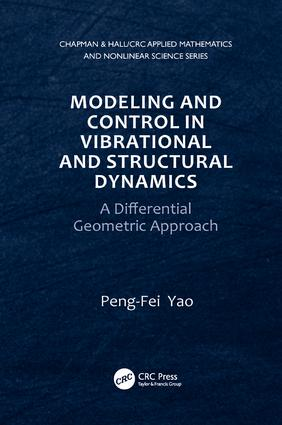# Modeling and Control in Vibrational and Structural Dynamics

## A Differential Geometric Approach, 1st Edition

CRC Press

419 pages | 2 B/W Illus.

##### Purchasing Options:\$ = USD
Paperback: 9781138116641
pub: 2017-06-07
SAVE ~\$16.59
\$82.95
\$66.36
x
Hardback: 9781439834558
pub: 2011-07-06
SAVE ~\$44.00
\$220.00
\$176.00
x
eBook (VitalSource) : 9780429094644
pub: 2011-07-06
from \$39.98

FREE Standard Shipping!

### Description

Modeling and Control in Vibrational and Structural Dynamics: A Differential Geometric Approach describes the control behavior of mechanical objects, such as wave equations, plates, and shells. It shows how the differential geometric approach is used when the coefficients of partial differential equations (PDEs) are variable in space (waves/plates), when the PDEs themselves are defined on curved surfaces (shells), and when the systems have quasilinear principal parts.

To make the book self-contained, the author starts with the necessary background on Riemannian geometry. He then describes differential geometric energy methods that are generalizations of the classical energy methods of the 1980s. He illustrates how a basic computational technique can enable multiplier schemes for controls and provide mathematical models for shells in the form of free coordinates. The author also examines the quasilinearity of models for nonlinear materials, the dependence of controllability/stabilization on variable coefficients and equilibria, and the use of curvature theory to check assumptions.

With numerous examples and exercises throughout, this book presents a complete and up-to-date account of many important advances in the modeling and control of vibrational and structural dynamics.

Preliminaries from Differential Geometry

Linear Connections, Differential of Tensor Fields, and Curvature

Distance Functions

A Basic Computational Technique

Sobolev Spaces of Tensor Field and Some Basic Differential Operators

Control of the Wave Equation with Variable Coefficients in Space

How to Understand Riemannian Geometry as a Necessary Tool for Control of the Wave Equation with Variable Coefficients

Geometric Multiplier Identities

Escape Vector Fields and Escape Regions for Metrics

Exact Controllability. Dirichlet/Neumann Action

Smooth Controls

A Counterexample without Exact Controllability

Stabilization

Transmission Stabilization

Control of the Plate with Variable Coefficients in Space

Multiplier Identities

Escape Vector Fields for the Plate

Exact Controllability from Boundary

Controllability for Transmission of Plate

Stabilization from Boundary for the Plate with a Curved Middle Surface

Linear Shallow Shells: Modeling and Control

Equations in Equilibrium. Green’s Formulas

Ellipticity of the Strain Energy of Shallow Shells

Equations of Motion

Multiplier Identities

Escape Vector Field and Escape Region for the Shallow Shell

Observability Inequalities. Exact Controllability

Exact Controllability for Transmission

Stabilization by Linear Boundary Feedbacks

Stabilization by Nonlinear Boundary Feedbacks

Naghdi’s Shells: Modeling and Control

Equations of Equilibrium. Green’s Formulas. Ellipticity of the Strain Energy. Equations of Motion

Observability Estimates from Boundary

Stabilization by Boundary Feedback

Stabilization of Transmission

Koiter’s Shells: Modeling and Controllability

Equations of Equilibria. Equations of Motion

Uniqueness for the Koiter Shell

Multiplier Identities

Observability Estimates from Boundary

Control of the Quasilinear Wave Equation in Higher Dimensions

Boundary Traces and Energy Estimates

Locally and Globally Boundary Exact Controllability

Boundary Feedback Stabilization

Structure of Control Regions for Internal Feedbacks

References

Bibliography

Index

Notes and References appear at the end of each chapter.

### Subject Categories

##### BISAC Subject Codes/Headings:
MAT000000
MATHEMATICS / General
MAT003000
MATHEMATICS / Applied
TEC007000
TECHNOLOGY & ENGINEERING / Electrical
TEC009070
TECHNOLOGY & ENGINEERING / Mechanical